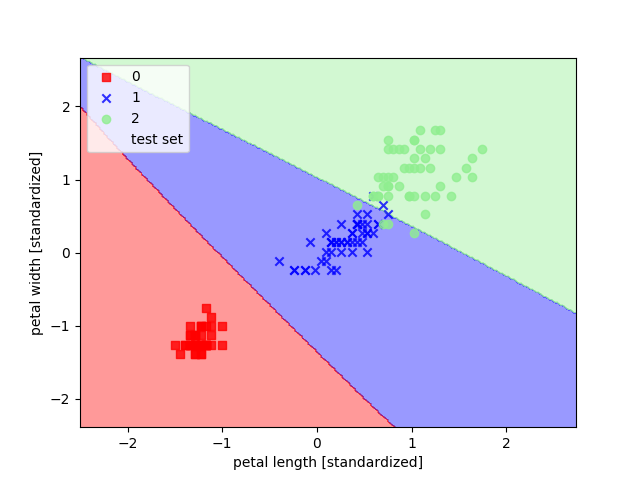# Example report with basic BookFlow¶

This is an example of a document that automatically updates when you execute new code runs. This updating happens when you build your book.

What you see on this page by default is only the output, the way you would want a document to show up like. However, you will also see, for the purposes of this example, buttons marked ‘click to show’, which will show you the code (python and markdown) that was used to enable this auto-updating from runs.

You see that the basic code is quite straightforward. Even so, I’m working on a set of helper utilities to make it even smoother, you can take a sneak peak on the next page.

The primary thing that you need to do with Jupyter Book is called ‘glueing’. By glueing a python object, you can insert it anywhere within your document by reference to the name provided in the glue() command. Note that these references are at the scope of the entire ‘book’, so you can glue something in one notebook, but then print it in another.

In this example, you can see examples of figures, tables and in-line variables (both strings and numeric). In order to learn how to include these, you can press some of the ‘click to show’ buttons, or follow the Jupyter Book documentation.

```%autosave 0
import mlflow
from myst_nb import glue
import pandas as pd

mlflow.set_tracking_uri('../iris_project/mlruns')
experiment_name = 'iris'
exp_id = mlflow.get_experiment_by_name(experiment_name).experiment_id

latest_run = mlflow.get_run(
mlflow.search_runs(
experiment_ids=[exp_id], max_results=1, filter_string="tags.valid='True'"
).loc.run_id
)

glue('algo', latest_run.data.params['algo'])
glue('acc', latest_run.data.metrics['acc_test'])

param_df = pd.DataFrame(latest_run.data.params.items(), columns=['Parameter','Value'])

glue('params', param_df, display=False)
```

## Methods¶

We have trained a svc model on the Iris dataset. The parameters used for this model can be found in this table.

```We have trained a {glue:text}`algo` model on the Iris dataset. The parameters used for this model can be found in {ref}`this table<param_table>`.
```
Parameter Value
0 gamma 0.1
1 C 1.0
2 algo svc
3 random_state 0
4 kernel rbf

Fig. 1 The values used for the parameters when training our svc model.

``````{glue:figure} params
:name: param_table
:figwidth: 300px

The values used for the parameters when training our {glue:text}`algo` model.
```
```

## Results¶

We achieve an accuracy of 0.96 on the test data. The decision boundaries can be seen in Fig. 2.

```We achieve an accuracy of {glue:text}`acc:.2f` on the test data. The decision boundaries can be seen in {numref}`decision_region`.
```
```from PIL import Image
im = Image.open(f'../iris_project/mlruns/{exp_id}/{latest_run.info.run_id}/artifacts/figures/decision_region.png')
glue('pil_image', im, display=False)
```Fig. 2 Here we show the decision regin of our svc model.

``````{glue:figure} pil_image
:name: decision_region

Here we show the decision regin of our {glue:text}`algo` model.
```
```
```import time

commit_hash = latest_run.data.tags.get('mlflow.source.git.commit', 'N/A')
run_id = latest_run.info.run_id
run_end_time = time.strftime('%Y-%m-%d %H:%M:%S', time.localtime(latest_run.info.end_time/1000.))
internal_meta_data = dict(
commit_hash=commit_hash,
run_id = run_id,
run_end_time = run_end_time
)

glue('int_meta_data', internal_meta_data)
```

## Appendix¶

```{'commit_hash': '91d164ec3550b45d9c4f4307aeadec3db495bb52',